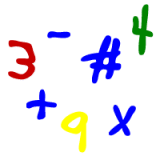## Roman NumeralsSkills needed:
Multiplication
Subtraction

Roman numerals were used by the Ancient Romans as their numbering system. We still use them sometimes today. You see them in the Super Bowl's numbering system, after king's names (King Henry IV), in outlines, and other places. Roman numerals are base 10 or decimal, like the numbers we use today. They are not entirely positional, however, and there is no number zero.

Roman numerals use letters instead of numbers. There are seven letters you need to know:
• I = 1
• V = 5
• X = 10
• L = 50
• C = 100
• D = 500
• M = 1000
You put the letters together to make numbers. Here are a few simple examples:

1) III = 3

three I's together is three 1's and 1 + 1 + 1 equals 3

2) XVI = 16

10 + 5 + 1 = 16

Sentence

1 I take my lunch box (Adrián)
1 I take my III lunch box (Carolina)
2 l  take my pencil (Miranda)
2 I take my IV Pencil case (Luciano)

,

#### 1 comentario:

1.Is small but is very cute